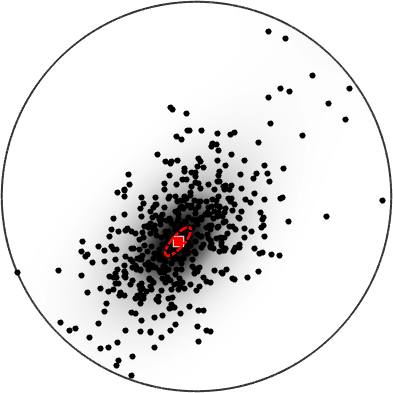The Spherical Bingham Distribution edit page

The Bingham distribution on the sphere is an antipodal symmetric distribution (Bingham, 1974) with a probabiliy density function given by

$p_{b}(\hat{x}\vert AKA^T) = \frac{1}{F(\kappa_{1},\kappa_{2},\kappa_{3})}\exp (\hat{x}^T AZA^T \hat{x})$

where $$A$$ is an orthognal covariance matrix, and $$Z$$ a concentration matrix with $$\mathrm{diag}(\kappa_{1},\kappa_{2},\kappa_{3})$$ with $$\kappa_{1} < \kappa_{2} < \kappa_{3}$$.

In mtex $$Z$$ is given by Z = [k1,k2,k3] with k3 = 0 and $$A$$ is given by three orthognal vectors.

Bingham, C., An Antipodally Symmetric Distribution on the Sphere, The Annals of Statistics Vol. 2, No. 6 (Nov., 1974), pp. 1201-1225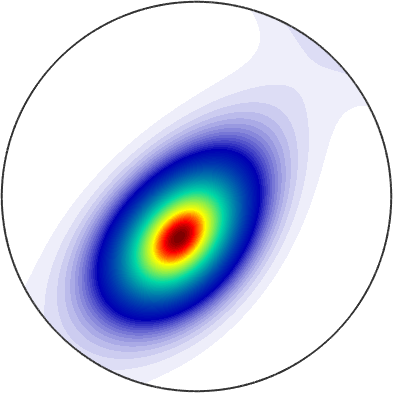## Meaning of $$Z$$

$$k1 = k2$$ defines a rotationally symmetric point maximum and $$k2 = 0$$ defines a girdle distribution.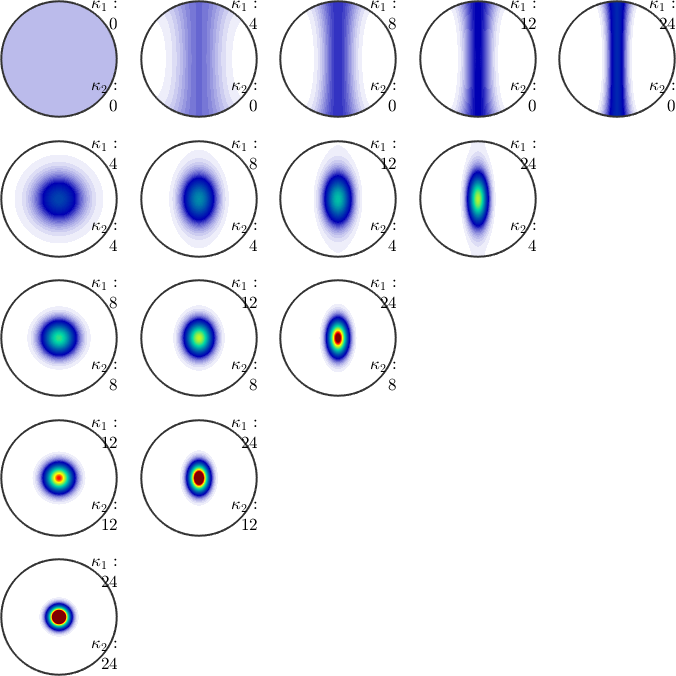## Drawing a random sample of the Bingham distribution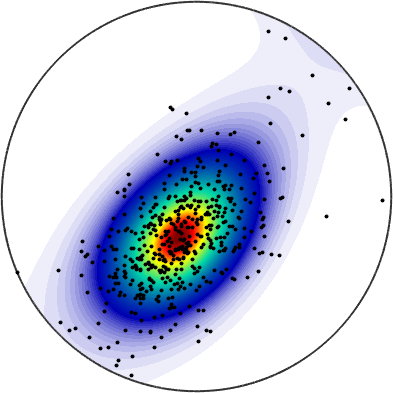## Estimating a spherical Bingham distribution from discrete data

Given arbitrarily scattered data v on the sphere we can estimate the best fitting Bingham distribution by

Lets plot the fitted distribution with the data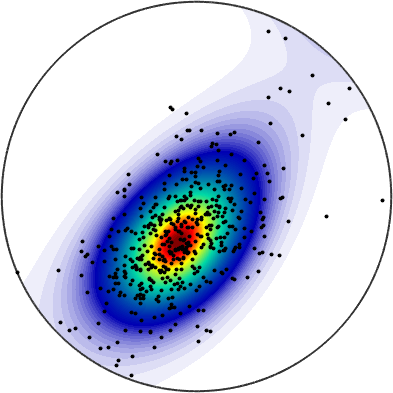Under the assumption of sufficiently many and sufficently concetrated data we may also estimate a confidence ellipse for the mean direction (default p = 0.95). The center of the ellipse is given by the largest principle vector stored in bs.a(3)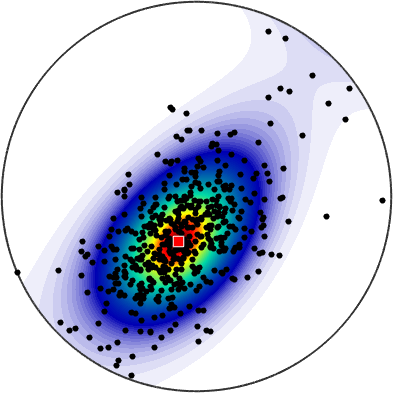The orientation of the ellipse is specified by all the principle vectors bs.a and the a and b axes are computed by the command cEllipse